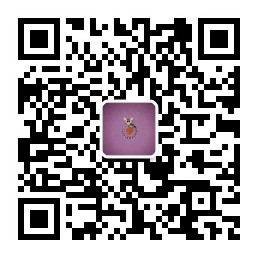Info

### # 一、twoSum 问题

``````vector<int> twoSum(vector<int>& nums, int target) {
// 先对数组排序
sort(nums.begin(), nums.end());
// 左右指针
int lo = 0, hi = nums.size() - 1;
while (lo < hi) {
int sum = nums[lo] + nums[hi];
// 根据 sum 和 target 的比较，移动左右指针
if (sum < target) {
lo++;
} else if (sum > target) {
hi--;
} else if (sum == target) {
return {nums[lo], nums[hi]};
}
}
return {};
}
``````

`nums` 中可能有多对儿元素之和都等于 `target`，请你的算法返回所有和为 `target` 的元素对儿，其中不能出现重复

``````vector<vector<int>> twoSumTarget(vector<int>& nums, int target);
``````